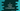# C program to convert a string to hexadecimal value## C program to convert a string to hexadecimal value:

In this post, we will learn how to convert a string to hexadecimal string in C programming language. Hexadecimal number system uses 16 as the base. 0 to 9 are used to represent decimal 0 to 9 and A, B, C, D, E, F are used to represent values from 10 to 15 in decimal. Lower case letters a, b, c, d, e, f are also used.

In many languages, 0x is also used as prefix for hexadecimal values.

For example, 0x3AB8 is a valid hexadecimal value. But 0xZXY is not.

### C program to do the conversion:

In this post, we will learn how to convert a string to hexadecimal in C. We will use a character array to hold the string. The conversion will convert each character of the string to hexadecimal and print out the final hexadecimal string.

For example, if the string is abc, it will:

So, the final hexadecimal converted string of abc will be 414243.

### Algorithm to follow:

We will follow the below algorithm to solve this issue:

• Ask the user to enter a string.
• Read the string and store it in a character array.
• Iterate through the characters of the array one by one.
• Convert each character to hexadecimal and store that value in a separate array.
• Print out the final hexadecimal string.

### Converting a character to hexadecimal value:

We will take help of the %x modifier. This modifier is used to convert to hexadecimal unsigned integer value. We can use %x for lowercase characters and %X for uppercase characters. For example, %x is for a,b,c etc. and %X is for A,B,C etc.

Let’s take a look at the below program:

``````#include <stdio.h>

int main()
{
printf("%x\n", 'z');
printf("%X\n", 'z');

return 0;
}``````

It will print:

``````7a
7A``````

As you can see here, %x is printing the hexadecimal value in lowercase and %X is printing the hexadecimal value in uppercase.

### Using sprintf:

We neet to use sprintf. sprintf is used for string print. We can use it to print data to a character array instead of a terminal.

• Read the user input string and store it in a character array
• Initialize one more character array to hold the output result.
• Iterate through the characters of the array one by one. For each character, use sprintf to write it to the final result array.
• Once the iteration is completed, print out the result array.

## C program:

Below is the complete program:

``````#include <stdio.h>
#include <string.h>

int main()
{
char givenStr, hexStr;

int i, j = 0;

printf("Enter a string: ");
scanf("%[^\n]s", givenStr);

for (i = 0; i < strlen(givenStr); i++)
{
sprintf(hexStr + j, "%02X", givenStr[i]);
j += 2;
}

hexStr[j] = '\0';

return 0;
}``````

Here,

• givenStr and hexStr are two character arrays and these are used to store the user given string and converted hexadecimal strings.
• i and j are two integers to use in the loop. These are initialized as 0 at the start of the program.
• It is reading the string as input from the user and storing this value in givenStr variable.
• The for loop runs through the characters of the string givenStr one by one and using sprintf, it is converting the character to hexadecimal value and inserting it in the character array hexStr. The %02 ensures that at least two values are created i.e. A will be 0A, 2 will be 02 etc.
• Once the loop ends, enter a \0 at the end.
• Print the hexadecimal string at the end.

If you run this program, it will give output as like below:

``````Enter a string: hello

Enter a string: a

Enter a string: world

## C program by using a different method:

We can also use a separate method to do the same thing. We can pass the strings or character arrays to a separate method and the method will do the processing.

``````#include <stdio.h>
#include <string.h>

void strToHexa(char *givenStr, char *hexStr)
{
int i, j = 0;
for (i = 0; i < strlen(givenStr); i++)
{
sprintf(hexStr + j, "%02X", givenStr[i]);
j += 2;
}

hexStr[j] = '\0';
}

int main()
{
char givenStr, hexStr;

printf("Enter a string: ");
scanf("%[^\n]s", givenStr);

strToHexa(givenStr, hexStr);